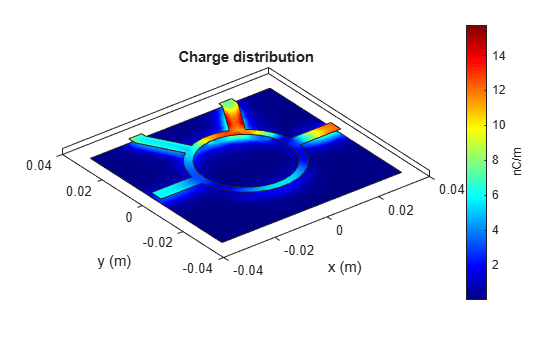# charge

Calculate and plot charge distribution

## Syntax

``charge(rfpcbobject,frequency)``
``c = charge(rfpcbobject,frequency)``
``[c,p] = charge(rfpcbobject,frequency)``
``charge(rfpcbobject,frequency,'dielectric')``
``c = charge(rfpcbobject,frequency,'dielectric')``
``c = charge(___,Name=Value)``

## Description

````charge(rfpcbobject,frequency)` calculates and plots the absolute value of the charge in C/m on the metal surface of a PCB component at the specified frequencies.```

example

````c = charge(rfpcbobject,frequency)` calculates a vector of charges in C/m on the metal surface of a PCB component, at the specified frequencies.```
````[c,p] = charge(rfpcbobject,frequency)` returns the point at which the charge calculation was performed.```
````charge(rfpcbobject,frequency,'dielectric')` calculates and plots the absolute value of the charge at the specified frequency on the dielectric surface of the PCB component.```
````c = charge(rfpcbobject,frequency,'dielectric')` calculates the charge on the dielectric surface of a PCB component at the specified frequency.```
````c = charge(___,Name=Value)` calculates the charge on the surface of a PCB component using additional name-value arguments.```

## Examples

collapse all

Create a rat-race coupler with default properties.

`coupler = couplerRatrace;`

Set the feed voltage and phase at the coupler ports.

`v = voltagePort(4)`
```v = voltagePort with properties: NumPorts: 4 FeedVoltage: [1 0 0 0] FeedPhase: [0 0 0 0] PortImpedance: 50 ```
`v.FeedVoltage = [1 0 1 0]`
```v = voltagePort with properties: NumPorts: 4 FeedVoltage: [1 0 1 0] FeedPhase: [0 0 0 0] PortImpedance: 50 ```
`v.FeedPhase = [90 0 270 0]`
```v = voltagePort with properties: NumPorts: 4 FeedVoltage: [1 0 1 0] FeedPhase: [90 0 270 0] PortImpedance: 50 ```

Calculate and view the charge distribution of the coupler at 3 GHz.

```figure charge(coupler,3e9,Excitation=v)```## Input Arguments

collapse all

PCB component object, specified as an RF PCB object. For a complete list of the PCB components and shapes, see PCB Components Catalog and Custom Geometry and PCB Fabrication.

Frequency to calculate charge distribution in hertz, specified as a scalar.

Example: 70e6

Data Types: `double`

### Name-Value Arguments

Example: `scale='log10'`

Scale to visualize the charge distribution on the surface of the PCB component, specified as a string or a character vector. The string values are either `'linear'`, `'log'`, or `'log10'` or as a function. You can specify any mathematical function such as `log`, `log10`, `cos`, or `sin`.

Data Types: `char` | `function_handle`

Excitation using as voltage source of N-ports to excite an N-port RF PCB component, specified a string or a function handle.

Data Types: `string` | `function_handle`

## Output Arguments

collapse all

Complex charges, returned as a 1-by-n vector in C/m. This value is calculated on every triangle mesh or every dielectric tetrahedron face on the surface of a PCB component.

Cartesian coordinates representing the center of each triangle in the mesh, returned as a 3-by-n real matrix.

## Version History

Introduced in R2021b